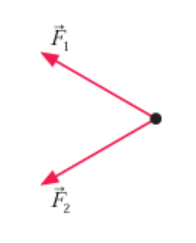# Problem: The figure (Figure 1) below shows two of the three forces acting on an object in equilibrium.Redraw the diagram, showing all three forces. Label the third force F3. F3⇀Draw the force vector with its tail at the dot. The orientation of your vectors will be graded. The exact length of your vectors will not be graded but the relative length of one to the other will be graded.

###### FREE Expert Solution

The y-components of F1 and F2 balance each other. Thus, the net force along the y-axis is zero.

The x-components of F1 and F2 add up.

Using Newton's second law:

ΣFx = - F1x - F2x + F3x = 0

F3x = F1x + F2x###### Problem Details

The figure (Figure 1) below shows two of the three forces acting on an object in equilibrium.Redraw the diagram, showing all three forces. Label the third force F3$\stackrel{\mathbf{⇀}}{{\mathbf{F}}_{\mathbf{3}}}$

Draw the force vector with its tail at the dot. The orientation of your vectors will be graded. The exact length of your vectors will not be graded but the relative length of one to the other will be graded.